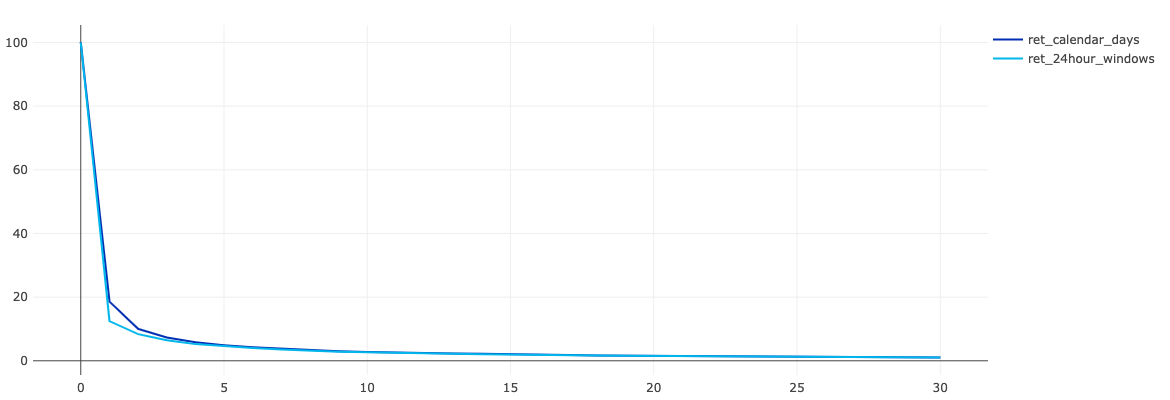# Calculating User Retention by 24-hour windows and calendar days in SQL

Время чтения текста – 3 минуты

Yesterday I got a message from one of our blog readers, asking:

Let’s say today’s Monday, and an app was downloaded 187 times. If I want to find out what’s the Retention rate was on day 1, what day of the week should I start taking the count with?

He is referring to the blog post on Сalculating Retention Rate. I would like to clarify that since retention rate can be calculated by 24-hour windows, as well as by calendar days. In our case, day 0 will be Monday, and day 1 will be Tuesday. However, there is one little hiccup...

For example, if we started promoting our product on Monday, October 12 at 23:59, then all downloads of this day will have the retention rate of day 1. It’s a problem of performing calendar calculations. To address this, some data analysts calculate retention rate not only by calendar days but also by 24-hour windows.

Let’s apply this idea to the above case:

• The Retention rate for day 0 can be calculated using the number of downloads from October 5, 23:59 to October 6, 23:59.
• The Retention rate for day 1: from October 6, 23:59 to October 7, 23:59
• And so on rolling 24-hour window.

## How to calculate Retention Rate by 24-hour windows in SQL?

Let’s recall one of the queries from our previous post. It was written to calculate the difference between the download date and the user activity date. We need to change the query so that user activity is calculated by 24-hour windows. To accomplish this just change the calculation for datediff to 24-hour windows, updating the lines in bold.

``````
SELECT from_unixtime(user.installed_at, "yyyy-MM-dd") AS reg_date,
floor((cast(cs.created_at as int)-cast(installed_at as int))/(24*3600)) as date_diff,
ndv(user.id) AS ret_base
FROM USER
LEFT JOIN client_session cs ON cs.user_id=user.id
WHERE 1=1
AND floor((cast(cs.created_at as int)-cast(installed_at as int))/(24*3600)) between 0 and 30
GROUP BY 1,2
``````
``` ```

Updated query:

``````SELECT
cohort.date_diff AS day_difference,
avg(reg.users) AS cohort_size,
avg(cohort.ret_base) AS retention_base,
avg(cohort.ret_base)/avg(reg.users)*100 AS retention_rate
FROM
(SELECT from_unixtime(user.installed_at, "yyyy-MM-dd") AS reg_date,
ndv(user.id) AS users
FROM USER
GROUP BY 1) reg
LEFT JOIN
(SELECT from_unixtime(user.installed_at, "yyyy-MM-dd") AS reg_date,
floor((cast(cs.created_at as int)-cast(installed_at as int))/(24*3600)) as date_diff,
ndv(user.id) AS ret_base
FROM USER
LEFT JOIN client_session cs ON cs.user_id=user.id
WHERE 1=1
AND floor((cast(cs.created_at as int)-cast(installed_at as int))/(24*3600)) between 0 and 30
GROUP BY 1,2
) cohort ON reg.reg_date=cohort.reg_date
GROUP BY 1
ORDER BY 1``````

## Final output:Compare it with the previous one:And as you see, the retention rate calculated using 24-hour windows is slightly lower in the first days.

Share
Send
Pin
601   2020   Q&A   redash   retention
Popular